# ISRO Scientist or Engineer Mechanical 2020

Instructions

For the following questions answer them individually

Question 51

# $$\cos(z)$$ can be expressed asQuestion 52

# Ina vector field; Divergence of the gradientisQuestion 53

# If a continuously differentiable vector function is the gradientof a scalar function, then its curl isQuestion 54

# What does ‘a’ and ‘e’ indicate in the surface texture symbol shown below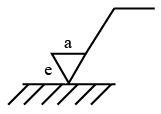Question 55

# Two steel trusses, XY and YZ of identical size supports a load of 200 N as shown in fig. The length of the truss is 1m. The force in the truss xy in N is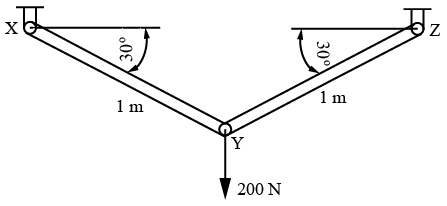Question 56

# A block weighing 100 N is resting on a plane inclined with horizontal as shown in Fig. What horizontal force P is necessary to hold the body from sliding down the plane? (Coefficient of friction can be taken as 0.25)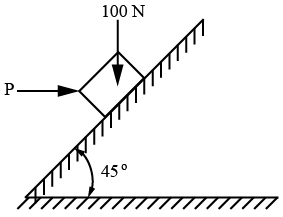Question 57

# The ratio of maximum shearstress to average shear stress in a beam ofrectangular section isQuestion 58

# A mass less beam has a loading pattern shown in figure. Find the bending moment at mid span?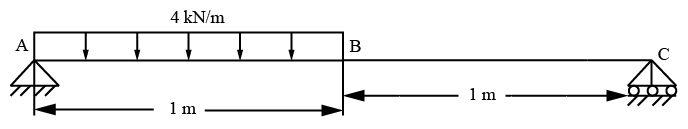Question 59

# Steel machine element at the critical section is in biaxial stress state with two principal stress being 300 $$\frac{N}{mm^{2}}$$ and 300 $$\frac{N}{mm^{2}}$$ (equal magnitude). Find the von Mises stress (in $$\frac{N}{mm^{2}}$$) in the memberQuestion 60

# A machine component of 90 kg mass needs to be held in position using three springs as shown below .The spring constants K_{1}, K_{2},K_{3} are 4,4 and 8 $$\frac{N}{m}$$ respectively. Find the natural freqeuncy of the system in $$\frac{rad}{sec}$$.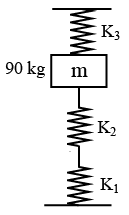OR